# Unit 5 Review Calculating area surface area and

• Slides: 51
Download presentationUnit 5 Review Calculating area, surface area and volumeWhat is the area?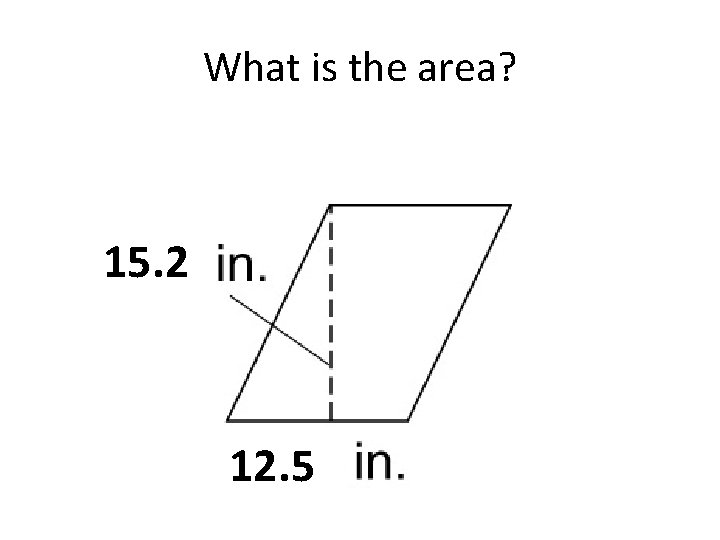What is the area? 15. 2 12. 5What is the area?What is the area? 6 7 12What is the area?What is the area? 6 3 7What is the area?What is the area?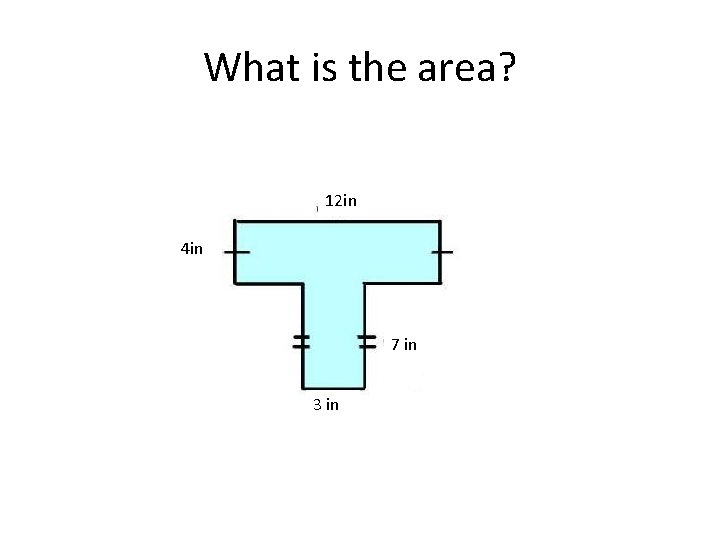What is the area? 12 in 4 in 7 in 3 inWhat is the area? 3 in 7 in 4 in 10 inCalculate the volume of this rectangular prism.Calculate the volume of this rectangular prism. 5 7 4Calculate the VolumeCalculate the Volume 12 8 8What is the surface area?What is the surface area?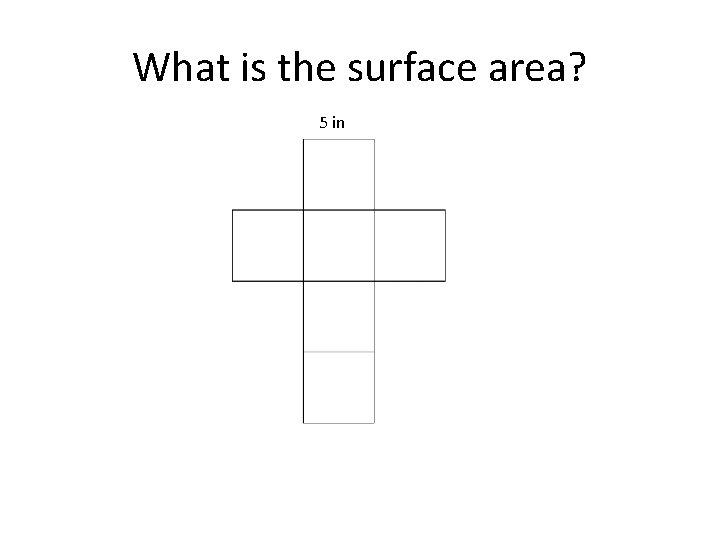What is the surface area? 5 inWhat is the surface area? 8 cm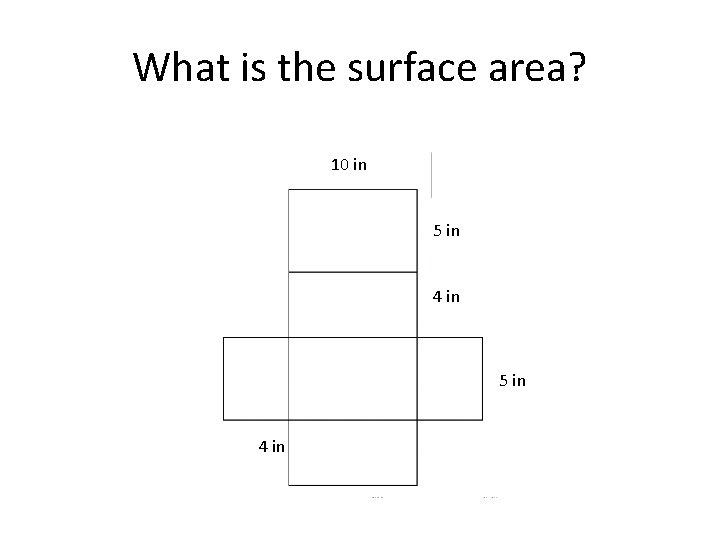What is the surface area? 10 in 5 in 4 in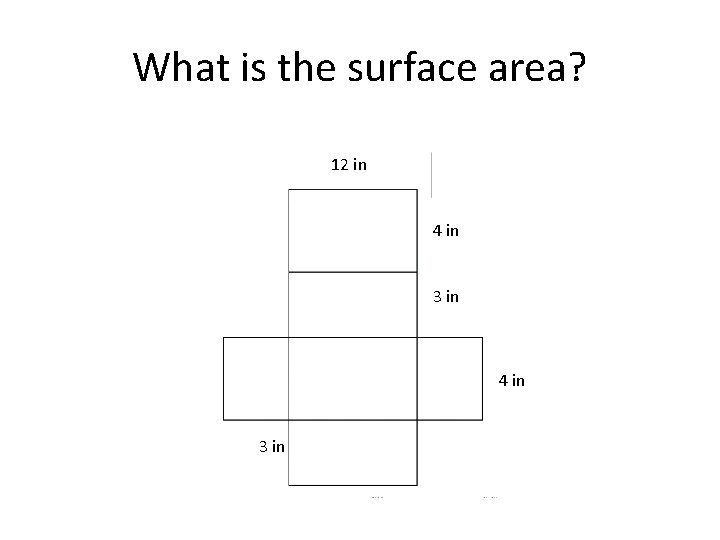What is the surface area? 12 in 4 in 3 in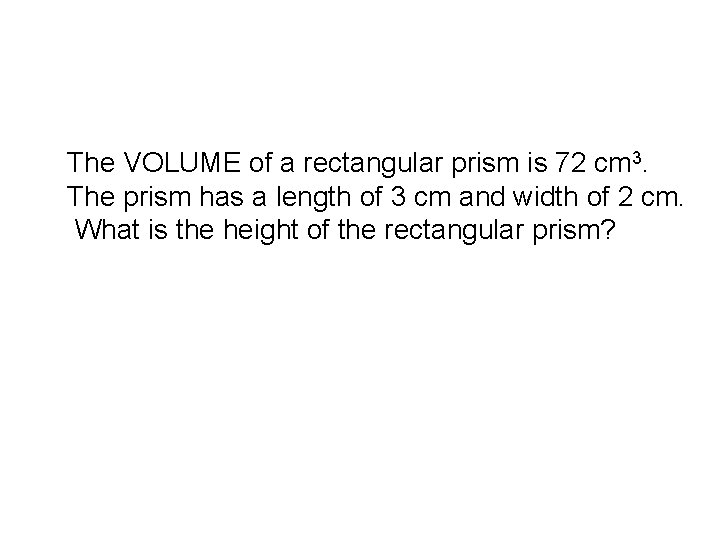The VOLUME of a rectangular prism is 72 cm 3. The prism has a length of 3 cm and width of 2 cm. What is the height of the rectangular prism?The VOLUME of a rectangular prism is 56 cm 3. The prism has a length of 4 cm and width of 2 cm. What is the height of the rectangular prism?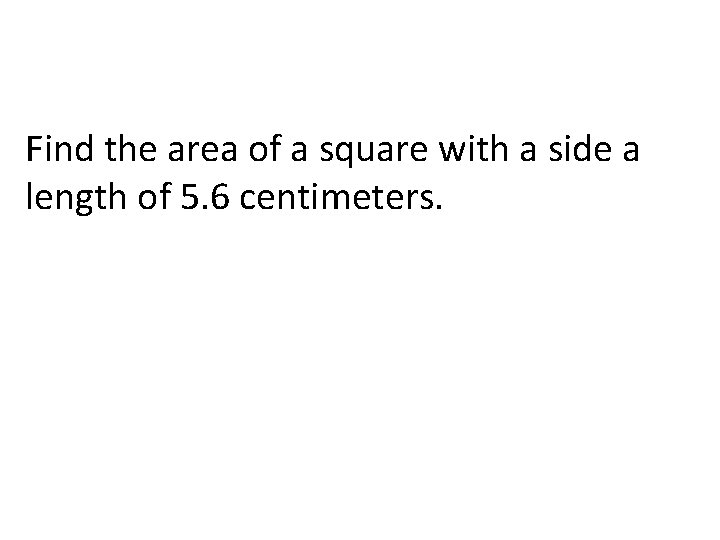Find the area of a square with a side a length of 5. 6 centimeters.Find the area of a square with a side a length of 7. 9 centimeters.Find the area of a rectangle that has a length of 12 meters and a width of 18 meters.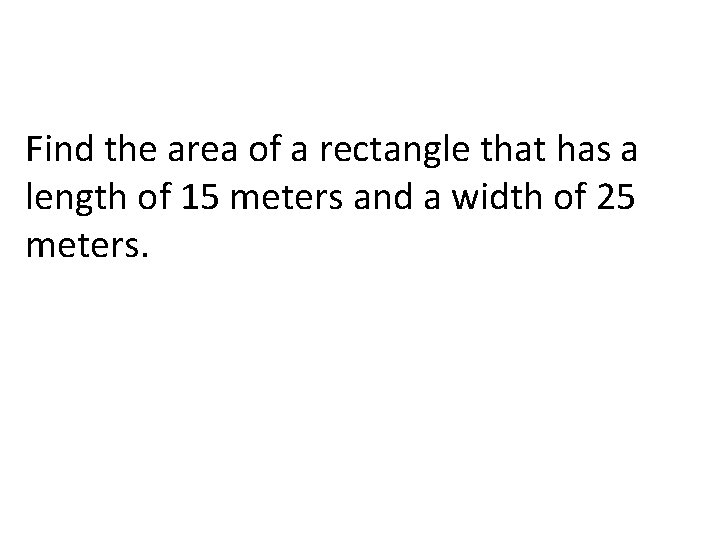Find the area of a rectangle that has a length of 15 meters and a width of 25 meters.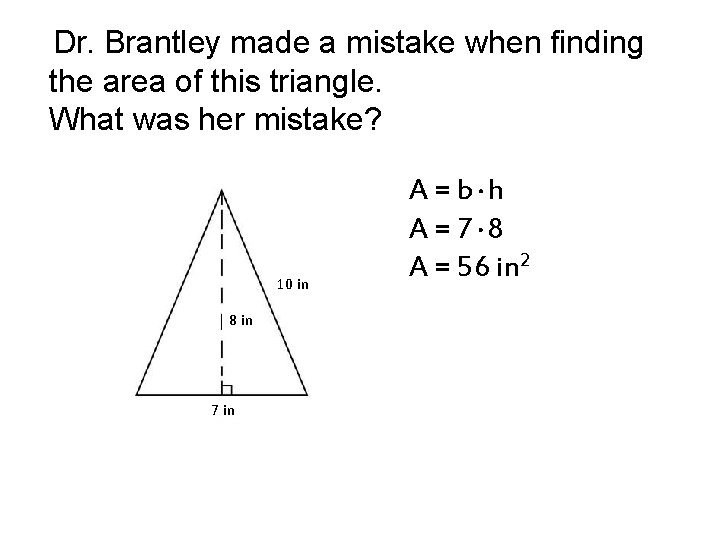Dr. Brantley made a mistake when finding the area of this triangle. What was her mistake? 10 in 8 in 7 in A = b. h A = 7. 8 A = 56 in 2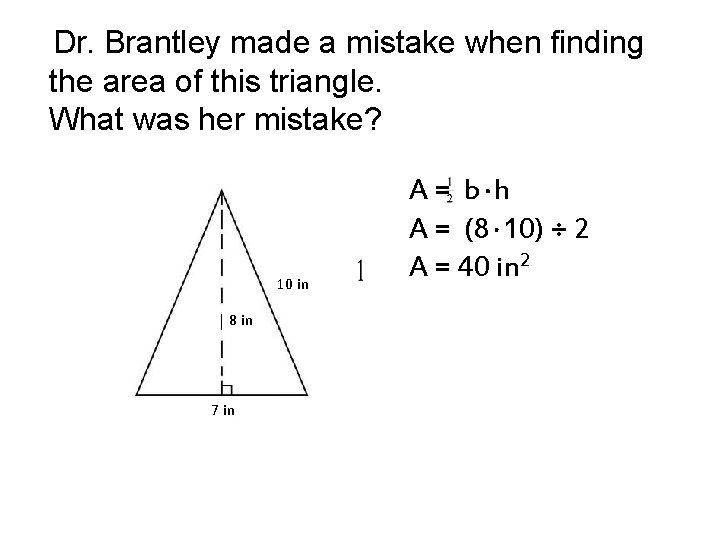Dr. Brantley made a mistake when finding the area of this triangle. What was her mistake? 10 in 8 in 7 in A = b. h A = (8. 10) ÷ 2 A = 40 in 2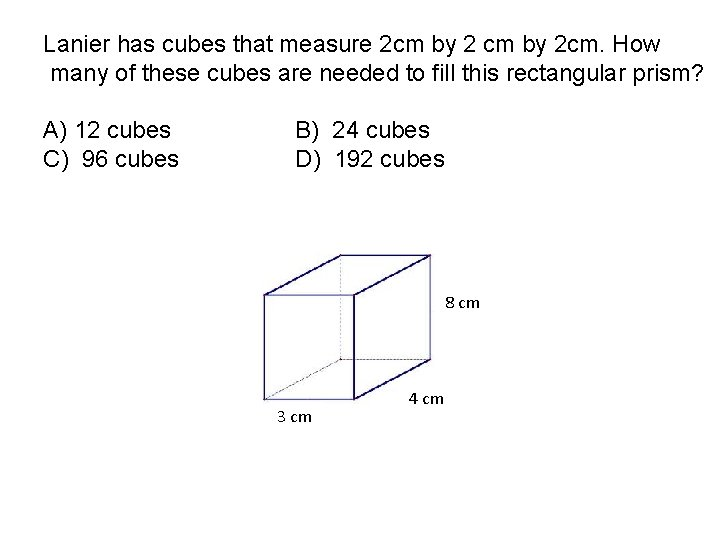Lanier has cubes that measure 2 cm by 2 cm. How many of these cubes are needed to fill this rectangular prism? A) 12 cubes C) 96 cubes B) 24 cubes D) 192 cubes 8 cm 3 cm 44 cm cmRuth has cubes that measure 2 cm by 2 cm. How many of these cubes are needed to fill this rectangular prism? A) 256 cubes C) 64 cubes B) 128 cubes D) 16 cubes 8 cm 44 cm cmJoey is packing a rectangular prism for his science teacher. The volume is 600 m 3. Which prism below CANNOT be Alex’s rectangular prism? A) B) C) D) 4 m by 25 m by 6 m 10 m by 6 m by 5 m by 20 m 10 m by 5 m by 10 m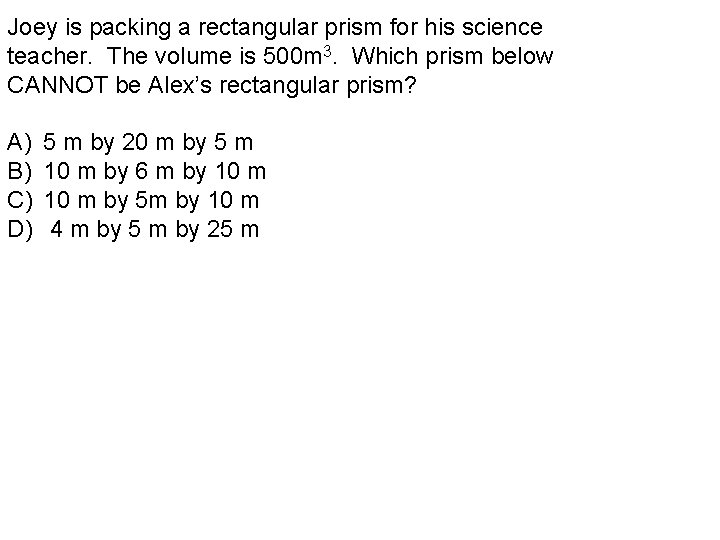Joey is packing a rectangular prism for his science teacher. The volume is 500 m 3. Which prism below CANNOT be Alex’s rectangular prism? A) B) C) D) 5 m by 20 m by 5 m 10 m by 6 m by 10 m by 5 m by 10 m 4 m by 5 m by 25 m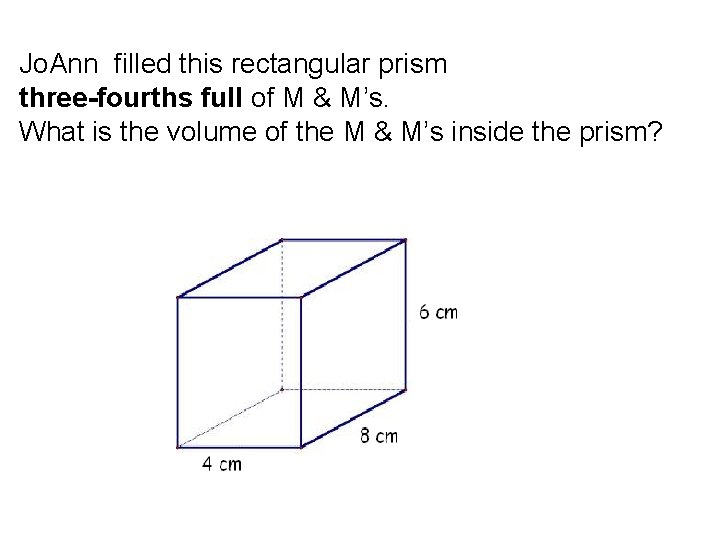Jo. Ann filled this rectangular prism three-fourths full of M & M’s. What is the volume of the M & M’s inside the prism?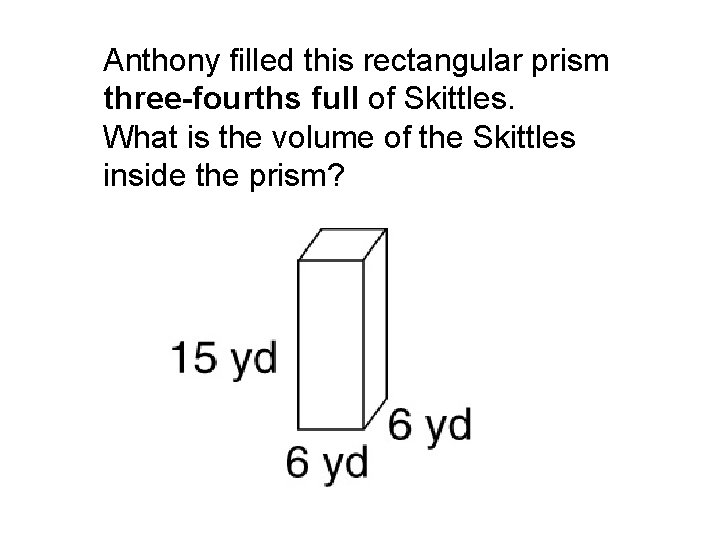Anthony filled this rectangular prism three-fourths full of Skittles. What is the volume of the Skittles inside the prism?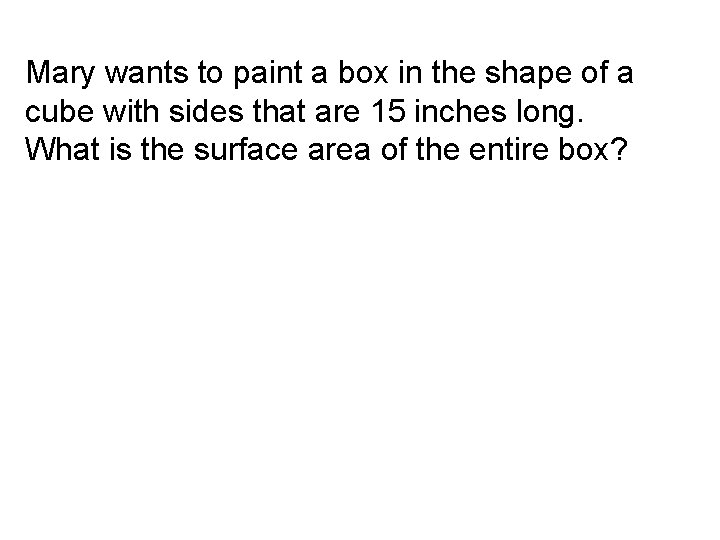Mary wants to paint a box in the shape of a cube with sides that are 15 inches long. What is the surface area of the entire box?Jessica wants to paint a box in the shape of a cube with sides that are 18 inches long. What is the surface area of the entire box?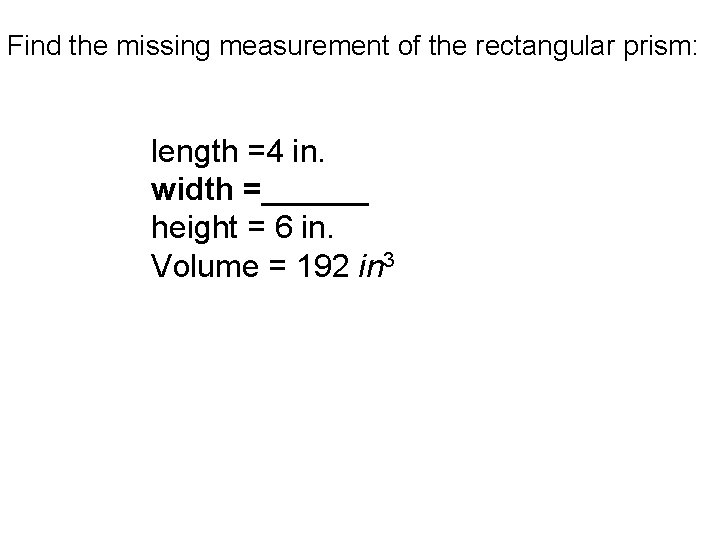Find the missing measurement of the rectangular prism: length =4 in. width =______ height = 6 in. Volume = 192 in 3Find the missing measurement of the rectangular prism: length =5 in. width =______ height = 8 in. Volume = 280 in 3You want to paint a jewelry box that is 12 inches long, 4 inches wide, and 7 inches high. Find the surface area of the jewelry box. Surface Area = ________ The label on the bottle of paint says the paint covers a total area of 345 square inches. Do you have enough paint to cover the entire box? Yes or No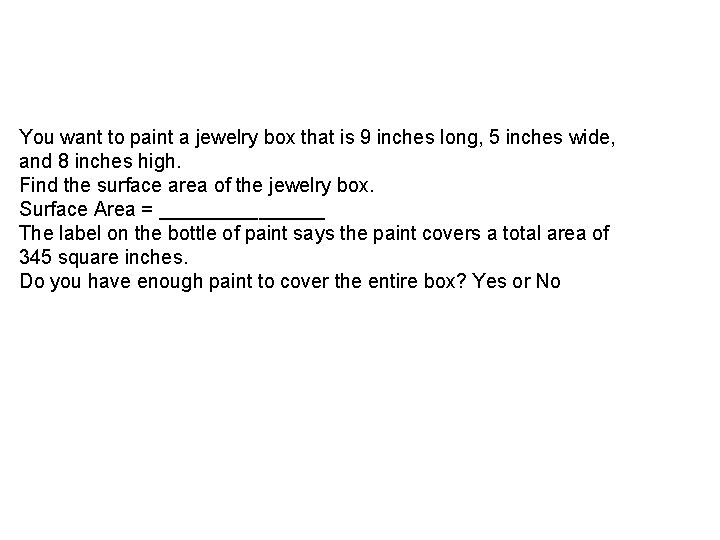You want to paint a jewelry box that is 9 inches long, 5 inches wide, and 8 inches high. Find the surface area of the jewelry box. Surface Area = ________ The label on the bottle of paint says the paint covers a total area of 345 square inches. Do you have enough paint to cover the entire box? Yes or No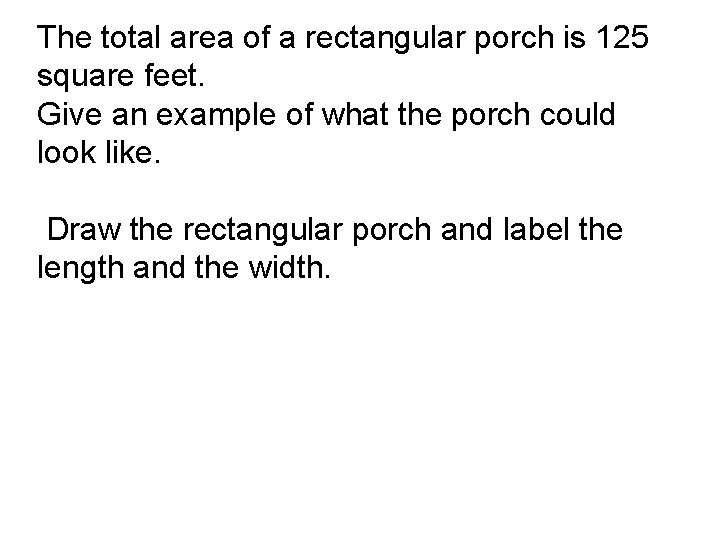The total area of a rectangular porch is 125 square feet. Give an example of what the porch could look like. Draw the rectangular porch and label the length and the width.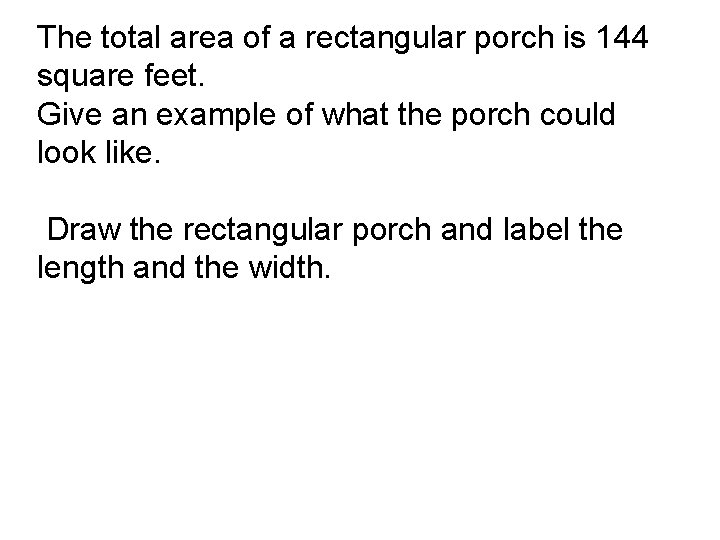The total area of a rectangular porch is 144 square feet. Give an example of what the porch could look like. Draw the rectangular porch and label the length and the width.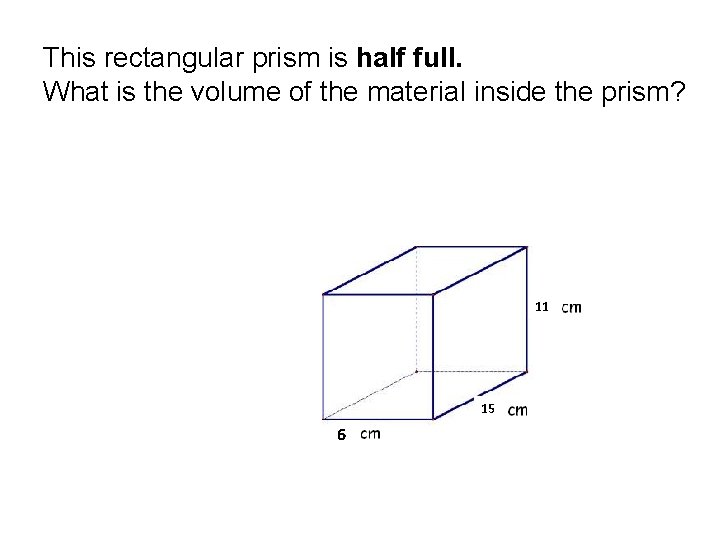This rectangular prism is half full. What is the volume of the material inside the prism? 11 15 6This rectangular prism is half full. What is the volume of the material inside the prism? 10 12 5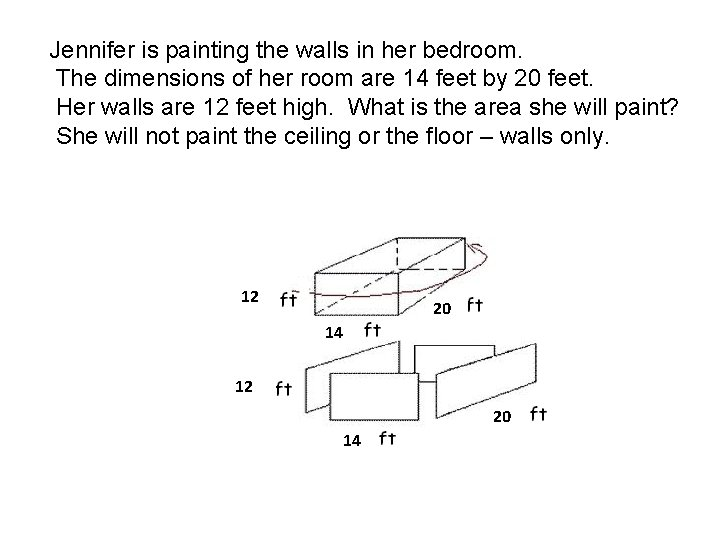Jennifer is painting the walls in her bedroom. The dimensions of her room are 14 feet by 20 feet. Her walls are 12 feet high. What is the area she will paint? She will not paint the ceiling or the floor – walls only. 12 20 14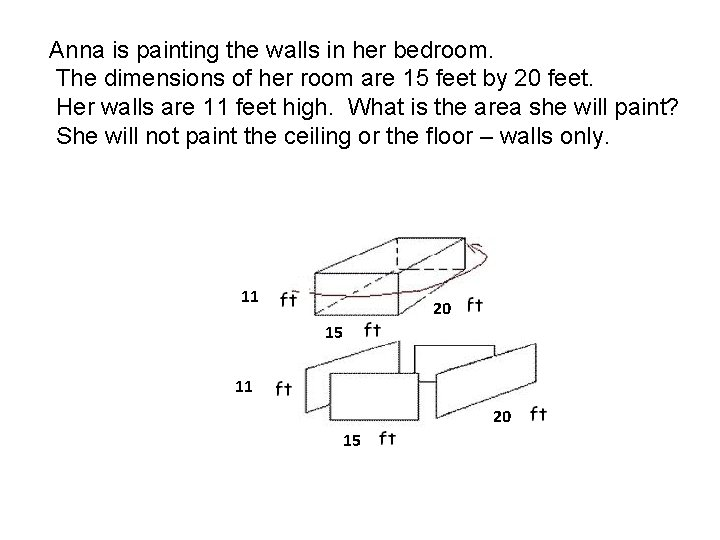Anna is painting the walls in her bedroom. The dimensions of her room are 15 feet by 20 feet. Her walls are 11 feet high. What is the area she will paint? She will not paint the ceiling or the floor – walls only. 11 20 15A rectangular storage container is 13 feet long, 12 feet wide and 10 feet high. What is the surface area of the storage container, including the ceiling and the floor?A rectangular storage container is 15 feet long, 12 feet wide and 12 feet high. What is the surface area of the storage container, including the ceiling and the floor?Find the surface area of the triangular prism. 4 cm 3 cm 4 cm 6 cm 2 cm 3 cm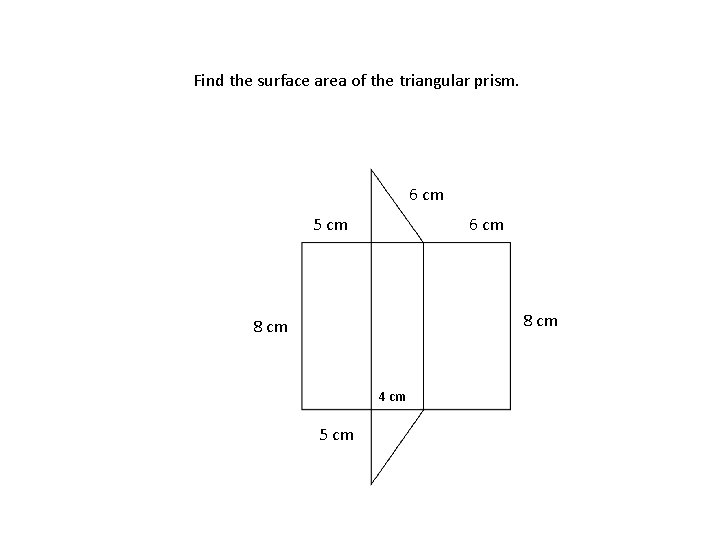Find the surface area of the triangular prism. 6 cm 5 cm 6 cm 8 cm 4 cm 5 cm